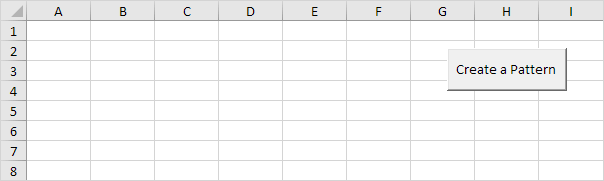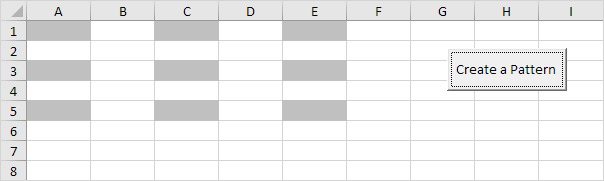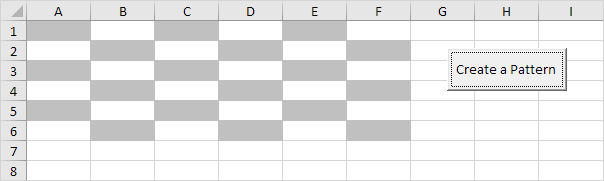# Create a Pattern

Below we will look at a program in Excel VBA that creates a pattern.

Situation:Place a command button on your worksheet and add the following code lines:

1. First, we declare two variables of type Integer. One named i and one named j.

Dim i As Integer, j As Integer

2. Second, we add two For Next loops.

For i = 1 To 5 Step 2
For j = 1 To 5 Step 2

3. Next, we add the line which changes the background color of the cells to light gray.

Cells(i, j).Interior.ColorIndex = 15

Note: instead of ColorIndex number 15 (light gray), you can use any ColorIndex number.

4. Close the two For Next loops.

Next j
Next i

5. Test the program.

Result so far.For example, for i = 1 and j = 1, Excel VBA colors Cells(1,1), for i = 1 and j = 3 (Step 2), Excel VBA colors Cells(1,3), for i = 1 and j = 5, Excel VBA colors Cells(1,5), for i = 3 (Step 2) and j = 1, Excel VBA colors Cells(3,1), etc.

6. We are almost there. The only thing we need to do, is color the cells which are offset by 1 row below and 1 column to the right of the cells already colored. Add the following code line to the loop.

Cells(i, j).Offset(1, 1).Interior.ColorIndex = 15

7. Test the program.

Result:Go to Next Chapter: Macro Errors